# Qlik Sense Documents

Qlik Sense documentation and resources.

Announcements
An issue has been identified on Qlik Cloud hub, please visit our Status Update Page for details: GET THE LATEST

# How to Design Simple Linear Regression in Qlik Sense with help of Microsoft R(R Integration with Qlik Sense )

cancel
Showing results for
Did you mean: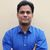Master

## How to Design Simple Linear Regression in Qlik Sense with help of Microsoft R(R Integration with Qlik Sense )

Hi Guys,

Recent days, I have been exploring scope of R(R is a programming language and free software environment for statistical computing and graphics supported by the R Foundation for Statistical Computing ) with Qlik Sense.

This article is next step of R Integration with Qlik Sense  which guide us how to install Microsoft R either on Same server or different and connect with Qlik Sense Enterprise or Qlik Sense Desktop.

Basic Description:

Qlik Sense Advanced Analytics integration is essentially an extension to Qlik Sense’s expression syntax, and as such it can be used in both Chart Expressions, and in Load Script Expressions.

With this new capability, we are now able to add syntax to a chart expression that tells Qlik Sense that particular expression should not be evaluated on the Qlik Sense server, but instead, all the information and data needed to calculate that expression should be sent via the server side extension on to the backend R system for calculation.

After the advanced analytic calculations are completed, the data is sent back to the Qlik Sense Server and to the client for visualization.

This article presumes R(either on Same server or different and connect with Qlik Sense Enterprise or Qlik Sense Desktop) is connected with Qlik Sense.

To Confirm the connection check RServe.exe CMD prompt: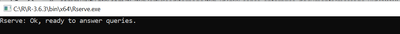SSEtoRserve.exe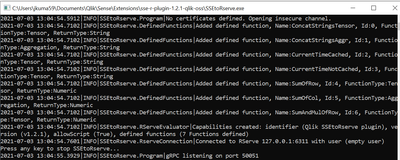If both consoles are showing running message it means we can start designing the Simple Linear Regression:

Lets discuss Simple Linear Regression,

In statisticssimple linear regression is a linear regression model with a single explanatory variable. That is, it concerns two-dimensional sample points with one independent variable and one dependent variable (conventionally, the x and y coordinates in a Cartesian coordinate system) and finds a linear function (a non-vertical straight line) that, as accurately as possible, predicts the dependent variable values as a function of the independent variable.

Y=b0 + b1*x1

Y is dependent variable

x is independent variable which means it is causing the change of value of Y(dependent variable)

b1 is  Coefficient which tells how much unit change in x1. Coefficient  could be multiple or divide means anyhow it if affecting the value of x1.

b0 is constant

Lets discuss a simple use case:

We have two columns in a simple sheet(same sheet is attached) YearsExperience and Salary and We are going to find what is the relation of Salary with YearsExperience.

Salary = b0  + b1*Experience

b0= when experience is 0 it means salary is 30k. it means when a person just joined the company, most probably that person has got 30k with no experience.

Red circle is b0 in  below image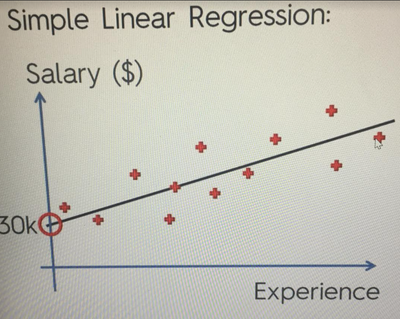If slope is increasing it means b1 is a positive number.

Black line is the linear regression line, we will find later what is best fit linear regression line.

Lets discuss this chart:

Red Plus signs + are the salary numbers which employees are earning and Black line which is a regressor line is what they should earnIt draws lots of possible lines and counts the sum of those squares every single time and it records it temporary  and then it finds the minimum value and that minimum value its Ordinary Least Squares Method. This is how simple linear regression works.

Sample data looks like

 YearsExperience Salary 1.1 39343 1.3 46205 1.5 37731 2 43525 2.2 39891 2.9 56642 3 60150

So on..

If we execute Linear regression formula in R studio:

regressor = lm(formula = Salary ~ YearsExperience,

data = training_set)

training set is the chuck of data on which we are running the Regressor.

First it tells you what formula is, the first line in below snapshot***(Three stars) tells the statistical significance. If No start means no statistical significance and if there are three stars *** means high statistical significance.

The lower of P value is means more impact of independent variable  on dependent variable. P value tells the possibility or significance.

If you visualise the above formula in R studio this is how it looks:

Blue line is Linear Regressor. It is the predictive salary.

lets do the same thing is Qlik Sense:

import Advance Analytics Extension into Qlik Sense

https://developer.qlik.com/garden/5979da222ef8975d99132f88

Open Qlik Sense=>Hub=>Create App=>

Drag and Drop down the extension:Select Simple Linear regression analysis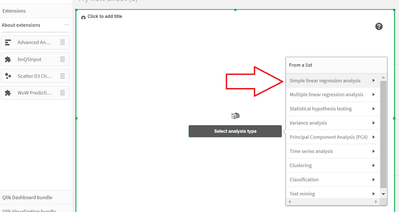Select Line chart with Linear Regression Line: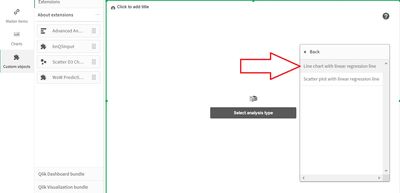Select YearsExperience as Dimension and Salary as Measure: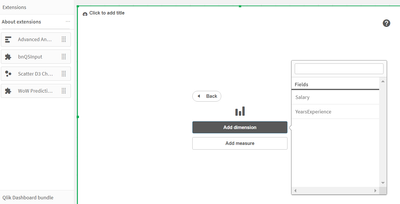Here is the outcome:

Qlik Sense has more advance graphic representation then R studio. It represents the formula, future predictive value, positive and negative area graph.Blue line is data actual points and red line is regressor line.

Data is same for both use cases, Representation in Qlik Sense has more impact than R, easy to create and manage.

Data appears in Qlik Sense but calculated in R.

Next regression model is : Multiple Linear Regression. Check out this document:

https://community.qlik.com/t5/Documents/How-to-Design-Multiple-Linear-Regression-in-Qlik-Sense-with-...

Reach out to me at kumar.rohit1609@gmail.com if there is need of any clarification or need assistance